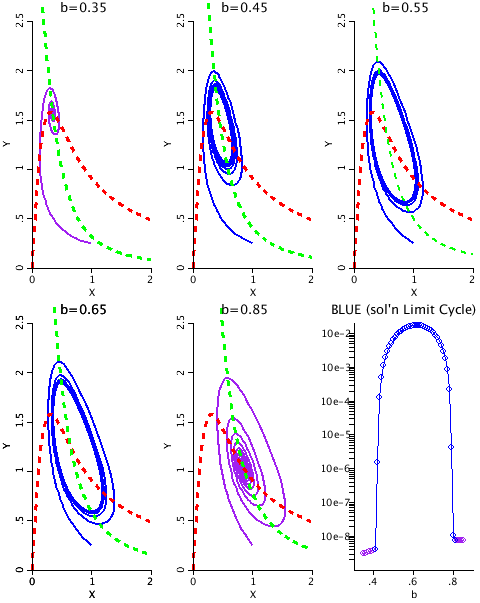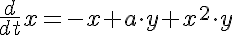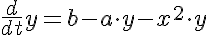# Selkov

Model number
0341

The Selkov model for glycolysis exhibits a Hopf bifurcation. As the b parameter increases from 0.25 to 0.95, the model switches from a stable equilibrium point to a limit cycle near b=0.41 and back to a stable equilibrium point near b=0.8.Figure 1: The phase plane plots showing oscillatory behavior.

## Description

This is a model showing oscillatory behavior in a chemical reaction with just two species.

PHASE PLANE PLOTS
Nullclines are plotted in dashed red (dx/dt=0) and dashed green (dy/dt=0).
Stable solutions are plotted in magenta (b=0.35, b=0.85)
Limit Cycles are plotted in blue (b=0.45, b=0.55, b=0.65).
Plow lower right corner: The standard deviation of the last half of the solutions were calculated for X and Y and the product, LC, was plotted as a function of b. Where LC is small, the solution has a stable steady state (Magenta circles). Where LC gets large, the solution is a limit cycle (Blue circles).

The appearance and disappearance of a periodic orbit through a local change in the stability properties of a steady point is known as a Hopf bifurcation. The Hopf bifurcations occur at approximately b=0.41 and b= 0.80 when a=0.1;

TIME COURSE PLOTS
There are 6 plots corresponding to 6 values of b. x is plotted in black, y in red.

Note that far away from the transition points the solutions converge rapidly (R=0.35, transition at 0.41). Close to the transition points the solutions converge more slowly (r=0.81, transition at 0.80). As the solution switches from stable solutions to Limit Cycles back to stable solutions, the size of the Limit cycles begins small, grows to a maximum and then shrinks back to zero. (r=0.42, r=0.55, r=0.65, and r=0.79).

## Equations

#### Selkov model equationsThe equations for this model may be viewed by running the JSim model applet and clicking on the Source tab at the bottom left of JSim's Run Time graphical user interface. The equations are written in JSim's Mathematical Modeling Language (MML). See the Introduction to MML and the MML Reference Manual. Additional documentation for MML can be found by using the search option at the Physiome home page.

References
```Selkov, E., Model of glycolytic oscillations, (1968) Eur. J. Biochem. 4, 79-86.

Strogatz, SH, Nonlinear Dynamics and Chaos, Addison-Wesley,
Reading, MA, (1994). (see pages 205-207)```
Key terms
glycolysis
Selkov
Hopf
bifurcation
phase plane